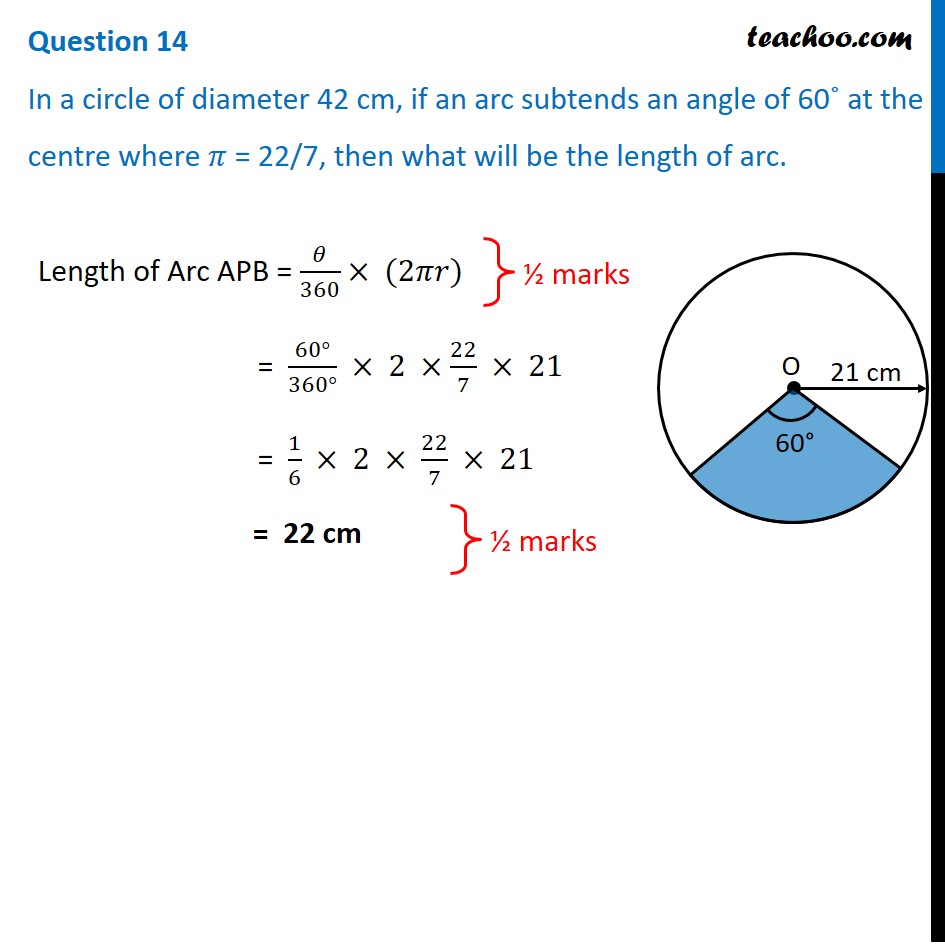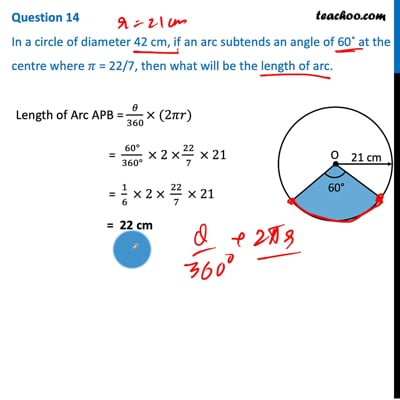CBSE Class 10 Sample Paper for 2021 Boards - Maths Standard

Class 10
Solutions of Sample Papers for Class 10 Boards

## In a circle of diameter 42cm,if an arc subtends an angle of 60˚ at the centre where π =22/7, then what will be the length of arc.This video is only available for Teachoo black users

Note : This is similar to Ex 12.2, 5 ( i ) of NCERT – Chapter 12 Class 10

Introducing your new favourite teacher - Teachoo Black, at only ₹83 per month

### Transcript

Question 14 In a circle of diameter 42 cm, if an arc subtends an angle of 60˚ at the centre where 𝜋 = 22/7, then what will be the length of arc.Length of Arc APB = 𝜃/360× (2𝜋𝑟) = (60°)/(360°) × 2 ×22/7 × 21 = 1/6 × 2 × 22/7 × 21 = 22 cm